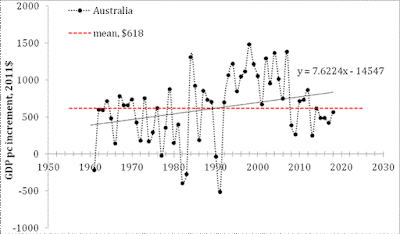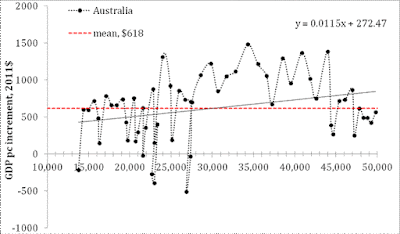## Growth Rate Of The GDP Per Capita Revisited

In this text devoted to the evolution of real GDP per capita, we present results of analysis based on the Maddison Project Database (MPD) data. The period of data coverage is 1960 to 2018. Since we have demonstrated the difference between the original (2008) and later (2012) studies based on the Total Economic Database data and the current dataset using Austria and the USA, we are not going to compare the MPD and TED data for other countries.

Figure 1 shows three plots for Australia. The upper panel depicts the annual GDPpc increment between 1961 and 2018 with the average value for the studied period of \$618 (2011 prices). The middle plot presents the same annual increment as a function of GDPpc level with the same mean value.

In the lower panel of Figure 1, the relative growth rate of the GDPpc is a function of the GDPpc, where the relative rate is the ratio of the GDPpc increment and the level at the beginning of the one year period, i.e. the growth rate in 1961 is [GDPpc(1961)-GDPpc(1960)]/GDPpc(1960). The straight average increment (red dashed) line in the middle panel is converted in the line \$618/GDPpc(t).

Australia demonstrates excellent (much above the average) economic growth between 1992 and 2007. The global recession reduced the rate of growth, but did not harm the growth much. The 2020 catastrophic fall induced by COVID-19 may also push the GDPpc growth rate in Australia below the zero line, and thus the slope of 0.115 \$/\$ in the middle panel may drop closer to the mean GDPpc line.

The Australian GDPpc level in 1960 was \$14013 (2011 prices). The beginning GDPpc value is an important parameter used in this study. Our model suggests that the total growth in a fixed period depends on the start value and the mean annual increment. For the same annual increment, a larger start value results in lower total growth.Figure 1. The upper panel: the annual GDPpc increment between 1961 and 2018 with the average value for the studied period of \$618 (2011 prices). The middle panel: the same annual increment as a function of GDPpc level. The lower panel: the relative growth rate of the GDPpc as a function of the GDPpc.

Disclosure: None.

How did you like this article? Let us know so we can better customize your reading experience. Users' ratings are only visible to themselves.